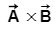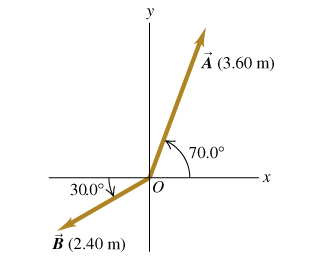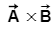# Problem: 1) For the two vectors,Find the magnitude of the vector product: Express your answer in square meters.2) What is the direction of the vector product: ?

###### FREE Expert Solution

1.

Vector components:

Cross product:

Magnitude:

$\overline{)\mathbf{|}\stackrel{\mathbf{⇀}}{\mathbit{A}}\mathbf{|}{\mathbf{=}}\sqrt{{{\mathbit{A}}_{\mathbit{x}}}^{\mathbf{2}}\mathbf{+}{{\mathbit{A}}_{\mathbit{y}}}^{\mathbf{2}}\mathbf{+}{{\mathbit{A}}_{\mathbf{z}}}^{\mathbf{2}}}}$

From vector components equation:

Ax = (3.60) cos (70°) = 1.23m i

Ay = (3.60) sin (70°) = 3.38m j

A = (1.23 i + 3.38 j) m

In a similar manner:

B = [(2.40) cos (180°+30.0°) i + (2.40) sin (180°+30.0°) j] m

B = (- 2.08 i - 1.2  j)m

The cross product:

A × B = (1.23 i + 3.38 j)×(- 2.08 i - 1.2  j)

87% (116 ratings)###### Problem Details

1) For the two vectors,

Find the magnitude of the vector product:2) What is the direction of the vector product:?